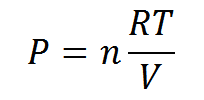Request a Tool

Daltons Law(Partial pressure) Calculator

The Dalton's law calculates gas flow in a solution.

Pressure
0

Formula• V = volume
• T = temperature
• Pi = partial pressure of the individual gas
• ni = number of moles of the gas
• R = ideal gas constant with a value of 8.314 J K-1 mol-1

Defination / Uses

Dalton's law of partial pressure measures total pressure using temperature, moles of gas and volume.

We measure gas pressure in daily life when we use a barometer to check the outside atmospheric pressure or a tires gauge to check the pressure in a bike tube. We're measuring a macroscopic physical property of a large number of gas molecules which aren't visible to the human eye when we perform this. The pressure we're measuring is the product of specific gas molecules colliding with other objects, such as the container's walls, at the molecular level.